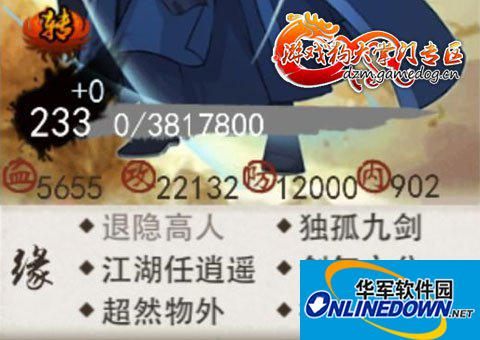# 大掌门潜力和血内的关系详细数据解析先普及一下基本概念：

(1)培养随机减的属性：精陪和普培都是-1 ，-2 或者 -3 ，随机加的属性：普培是+2 ，+3 ，+4 ，+ 5 ，+6，精培是 +3 ，+4 ， +5 ，+6 ，+7 ，+8 ，+9。

(2)特别注意：消耗潜力是有范围的，普培是消耗1至3潜力，精培是2至6。也就是说普培没有 (-1 + 5) 精培不会出现(-2 + 9)

(3)一次一次培养，非10培

一。普培时：消耗潜力和血内关系(攻防培养)

(1)纯攻防培养(接受攻防互转，不接受-血+内)：总共接受54种培养方案，具体如下

+攻-血/内/防：(潜力-1)-1+2，-2+3，-3+4;(潜力-2)-1+3，-2+4，-3+5;(潜力-3)-1+4，-2+5，-3+6，

+防-血/内/攻：(潜力-1)-1+2，-2+3，-3+4;(潜力-2)-1+3，-2+4，-3+5;(潜力-3)-1+4，-2+5，-3+6，

结论：假定出现概率一样的情况下，都各出现一次(9*3*2=54次)，那么消耗潜力=(1*3+2*3+3*3)*3*2=108，消耗的血内=(1*3+2*3+3*3)*2*2=72。即普培情况下，3点潜力减去2点血内。

(2)攻防偏攻或偏防培养(接受攻防单向互转，不接受-血+内)：总共接受45种培养方案，举例如下(攻防偏攻)

+攻-血/内/防：(潜力-1)-1+2，-2+3，-3+4;(潜力-2)-1+3，-2+4，-3+5;(潜力-3)-1+4，-2+5，-3+6，

+防-血/内：(潜力-1)-1+2，-2+3，-3+4;(潜力-2)-1+3，-2+4，-3+5;(潜力-3)-1+4，-2+5，-3+6，——这里注意，不接受+防-攻

结论：假定出现概率一样的情况下，都各出现一次(9*3+9*2=45次)，那么消耗潜力=(1*3+2*3+3*3)*3+(1*3+2*3+3*3)*2=90，消耗的血内=(1*3+2*3+3*3)*2*2=72。即攻防偏攻或偏防普培情况下，5点潜力减去4点血内。

二。精培时：消耗潜力和血内关系(攻防培养)

(1)纯攻防培养(接受攻防互转，不接受-血+内)：总共接受90种培养方案，具体如下

+攻-血/内/防：(潜力-2)-1+3，-2+4，-3+5;(潜力-3)-1+4，-2+5，-3+6;(潜力-4)-1+5，-2+6，-3+7;(潜力-5)-1+6，-2+7，-3+8;

(潜力-6)-1+7，-2+8，-3+9;

+防-血/内/攻：(潜力-2)-1+3，-2+4，-3+5;(潜力-3)-1+4，-2+5，-3+6;(潜力-4)-1+5，-2+6，-3+7;(潜力-5)-1+6，-2+7，-3+8;

(潜力-6)-1+7，-2+8，-3+9;

结论：假定出现概率一样的情况下，都各出现一次(3*5*3*2=90次)，那么消耗潜力=(2*3+3*3+4*3+5*3+6*3)*3*2=360，消耗的血内=(1*5+2*5+3*5)*2*2=120。即精培情况下，3点潜力减去1点血内。

(2)攻防偏攻或偏防培养(接受攻防单向互转，不接受-血+内)：总共接受75种培养方案，举例如下(攻防偏攻)

+攻-血/内/防：(潜力-2)-1+3，-2+4，-3+5;(潜力-3)-1+4，-2+5，-3+6;(潜力-4)-1+5，-2+6，-3+7;(潜力-5)-1+6，-2+7，-3+8;

(潜力-6)-1+7，-2+8，-3+9;

+防-血/内：(潜力-2)-1+3，-2+4，-3+5;(潜力-3)-1+4，-2+5，-3+6;(潜力-4)-1+5，-2+6，-3+7;(潜力-5)-1+6，-2+7，-3+8;

(潜力-6)-1+7，-2+8，-3+9;

结论：假定出现概率一样的情况下，都各出现一次(3*5*3+3*5 *2=75次)，那么消耗潜力=(2*3+3*3+4*3+5*3+6*3)*3+(2*3+3*3+4*3+5*3+6*3)*2=300，消耗的血内=(1*5+2*5+3*5)*2*2=120。即精培情况下，5点潜力减去2点血内。

三。实际应用

以小李为例，我洗髓前普培，接受-血+内，大致到180级，满培后，血大概1300-1400，内1150-1200左右，按最大的算，洗髓后血=1400-15*20-15*20=800，内=1200-5*20=1100

那么开始精培(攻防偏攻，只接受+攻-防)，那么根据上述描述，每5点潜力减去2点血内，即4000潜减去1600潜力，假若加血内概率一样，各减800，那么最后血为0，内估计300左右。